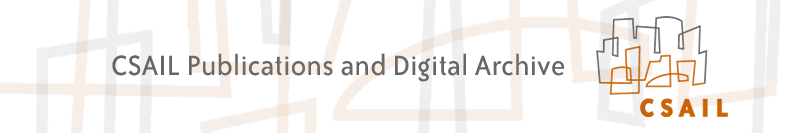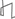Technical Reports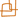Work Products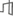Research Abstracts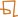Historical Collections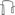Research Abstracts - 2006IntroductionLanguage, Learning, Vision & GraphicsPhysical, Biological & Social SystemsSystemsTheory### Lower Bounds for Dynamic Data Structures

#### Erik D. Demaine & Mihai Pǎtraşcu

##### What

We have developed a new technique for proving lower bounds for dynamic data-structure problems in the powerful cell-probe model of computation. Our technique is the first which can prove a lower bound of Ω(lg n) in this general model, breaking a long-standing barrier of Ω(lg n / lg lg n). Using this approach, we proved lower bounds for the partial-sums problem and the dynamic connectivity problem. Our lower bounds are either known or conjectured to be tight.

##### Why

When proving lower bounds for specific problems, a crucial point is what model of computation is assumed. Many lower bounds are proved in weak, structured models, and thus are not always relevant. For example, the progress made in the past decades in designing efficient data structures has broken most previous lower bounds for the comparison model or the pointer machine.

A very general model for analyzing data structures is the cell probe model. In this model, we assume that memory consists of b-bit words or cells, and only count the cell probes made by the algorithm (cell reads or writes). It is also assumed that b = Ω(lg n), where n is the size of the problem, so that natural quantities fit in a word. Since no restriction is made on the way data can be manipulated, this model is general enough, that lower bounds are relevant in any model of computation that is physically implementable today. In particular, the model is at least as strong as the pointer machine, the comparison model, or the more interesting Random Access Machine (RAM) model. In addition, by equating a ``cell'' with a ``page'' (and possibly having b >>lg n), the cell-probe model also captures the external memory and cache-oblivious models.

However, this great power leads to great challenges. Prior to our work, the only technique for proving lower bounds for dynamic data structures in the cell-probe model was the chronogram technique, introduced by Fredman and Saks in 1989 [FS89]. This technique is rather cumbersome to use, and only about three problems have been successfully analyzed using this approach (a few other bounds follow by reductions). In addition, the technique is inherently limited, and cannot prove a bound exceeding ω(lg n / lg lg n). Breaking this barrier has been considered one of the most important problems in data structures [Mil99].

##### How

Our technique begins by considering a random sequence of updates and queries (drawn according to a problem-specific distribution). Then, we consider a balanced tree (of appropriate branching factor) with the sequence of operations in the leaves—this can be visualized as a tree built over the time axis. A read instruction is determined by the time when it occurs, and the time when the location was last written. We associate every read instruction with a node in the lower-bound tree, corresponding to the longest common prefix between the update and read times. We then show that every node in the tree must have a large number of read instructions associated with it. Proofs of this fact involve elementary results from Kolmogorov complexity and clever encoding schemes. These encoding schemes are able to reconstruct all information from updates in a given subtree (which have high Kolmogorov complexity), based on the set of read instructions associated with a node. Thus we conclude that there must be many such instructions corresponding to that node.

Our approach is significantly more global that the chronogram method, and thus can prove stronger bounds. In addition, relying on clever encodings instead of complicated combinatorial arguments makes the proofs simpler.

##### Progress

Our main results in [PD06] are Ω(lg n) lower bounds for dynamic connectivity (answering connectivity queries in undirected graphs subject to updates) and for the dynamic partial-sums problem (sometimes known as the prefix-sums problem). We also obtained a number of additional results, such as lower bounds on the tradeoffs between update and query times, and Ω(lgB n) lower bounds for external-memory models. In nearly all cases, our bounds are either tight or conjectured to be tight.

##### Future

By far the most appealing open problem is to break the Ω(lg n) barrier established by our results. Natural candidate problems are dynamic range queries in higher dimensions, and dynamic k-connectivity. Applying our technique to other natural problems would also be quite interesting, even if the bounds do not exceed Ω(lg n).

It would be nice to obtain an O(lg n) upper bound for dynamic connectivity, closing this problem; the current bounds are O(lg n ⋅ poly(lg lg n)). It would also be interesting to prove that our lower bound holds in some restricted cases, such as grid graphs or decremental connectivity. Finally, our technique cannot obtain satisfactory tradeoff lower bounds when the query time exceeds the update time. We believe that fixing this restriction would be very interesting, but would probably require significant additional insight.

##### References
[FS89]
Michael L. Fredman and Michael E. Saks. The cell probe complexity of dynamic data structures. In Proceedings of the 21st ACM Symposium on Theory of Computing, pp. 345–354, 1989.
[Mil99]
Peter Bro Miltersen. Cell probe complexity — a survey. In Advances in Data Structures Workshop, Conference on the Foundations of Software Technology and Theoretical Computer Science, 1999.
[PD06]
Mihai Pǎtraşcu and Erik D. Demaine. Logarithmic lower bounds in the cell-probe model. SIAM Journal on Computing, to appear.Computer Science and Artificial Intelligence Laboratory (CSAIL) The Stata Center, Building 32 - 32 Vassar Street - Cambridge, MA 02139 - USA tel:+1-617-253-0073 - publications@csail.mit.edu